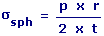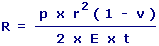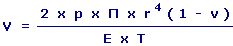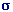Pressure Loading of Thin-walled Vessels (Sphere) Calculator Enter value and click on calculate. Result will be displayed.sph = Stress p = Uniform Internal Pressure r = Radius t = Thickness v = Poisson's Ratio E = Modulus of Elasticity R = Increase In Radius V = Increase In Volume
 Enter your values: Radius (r): m Thickness (t): m Modulus of Elasticity (E): 109 N / m2 Poisson's Ratio (v): Uniform Internal Pressure (p): 106 N / m2 Results: Stress (sph): 106 N / m2 Increase In Radius (R): 10-6 m Increase In Volume (V): 10-6 m3

 Web www.calculatoredge.com
 A thin-walled vessel is one where the thickness of the wall is no greater than one-tenth of the radius. Consider such a vessel subjected to an internal pressure above atmospheric pressure. The resulting stresses and expansion of the vessels are calculated by this calculator.

Poisson's ratio, named after Simeon Poisson, is the ratio of the relative contraction strain, or transverse strain (normal to the applied load), divided by the relative extension strain, or axial strain (in the direction of the applied load).

Stress is a measure of the average amount of force exerted per unit area. It is a measure of the intensity of the total internal forces acting within a body across imaginary internal surfaces, as a reaction to external applied forces and body forces.LINKSDISCLAIMERCONTACT US